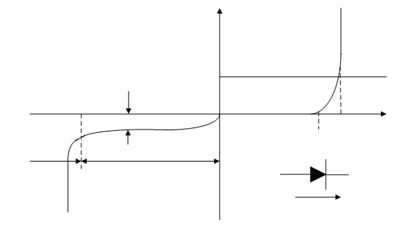## Characteristics of a Diode Assignment Help

Assignment Help: >> Diodes - Characteristics of a Diode

Characteristics of a Diode:

For forward bias case, current I becomes extremely much larger than I0 therefore the subtraction of I0 from I is negligible and I becomes I = I0 exp eV/KT  . For reverse bias, V is negative therefore the exponential term is very small and negligible therefore I ≈ I0 . Leakage current is typically few µA. At room temperature, T = 300K, e/ KT   = 40 therefore for forward bias, I ≈ I0  exp40V . For a linear resistor, R =V/I     is the relation between voltage and current across a resistor R, but here the relationship among V an I is not constant but exponential. Therefore the slope of the line at any point gives the "resistance" for forward bias junction.  dI /dV ≈ 40 I0  exp40V = 40 I , therefore dV /dI =   1/40 I = r = 25 mV/ I . R is called the dynamic or small signal resistance of the forward biased diode junction.Figure: I-V Characteristics of a Diode